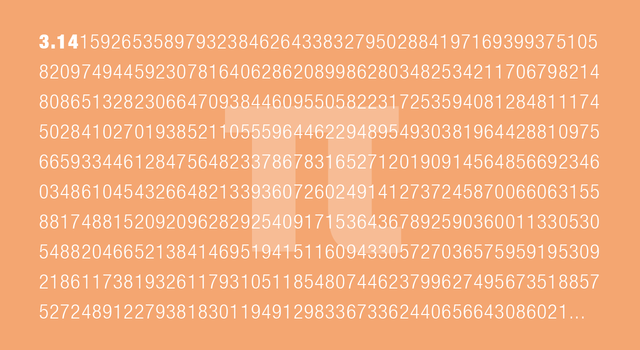You've probably seen many different methods of how to memorise the first 100 digits of pi, like this one or this one but have you ever thought how practically useful this would be? I have and so did a NASA-JPL fan recently.

I'll cut to the chase and say NASA-JPL only use the first 15 decimal places, ie 3.141592653589793.

Why? Well, they give three really good practical examples, with the first two being my favourite:

The most distant spacecraft from Earth is Voyager 1. It is about 12.5 billion miles away. Let's say we have a circle with a radius of exactly that size (or 25 billion miles in diameter) and we want to calculate the circumference, which is pi times the radius times 2. Using pi rounded to the 15th decimal, as I gave above, that comes out to a little more than 78 billion miles. We don't need to be concerned here with exactly what the value is (you can multiply it out if you like) but rather what the error in the value is by not using more digits of pi. In other words, by cutting pi off at the 15th decimal point, we would calculate a circumference for that circle that is very slightly off. It turns out that our calculated circumference of the 25 billion mile diameter circle would be wrong by 1.5 inches. Think about that. We have a circle more than 78 billion miles around, and our calculation of that distance would be off by perhaps less than the length of your little finger.

Wow!! Only an inaccuracy of 1.5 inches or about 3.8 centimetres out of a 78 billion miles!! That's pretty impressive. But wait, you're probably thinking that that's not really practical; who other than rocket scientists, need to calculate the circumference of a circle that big?

Well, that's where their second example comes in…

We can bring this down to home with our planet Earth. It is 7,926 miles in diameter at the equator. The circumference then is 24,900 miles. That's how far you would travel if you circumnavigated the globe (and didn't worry about hills, valleys, obstacles like buildings, rest stops, waves on the ocean, etc.). How far off would your odometer be if you used the limited version of pi above? It would be off by the size of a molecule. There are many different kinds of molecules, of course, so they span a wide range of sizes, but I hope this gives you an idea. Another way to view this is that your error by not using more digits of pi would be 10,000 times thinner than a hair!

Let me rephrase that last part for you: by using pi to the 15th decimal place to calculate the circumference of the earth, you'd be off by less than 10,000 times the thickness of a hair!! You wouldn't even be able to see the difference.

I'm sold. I don't care about impressing my friends with my memory, I'm going to concentrate on "just enough" accuracy like the NASA peeps do.

Now go and memorise this number: 3.141592653589793.